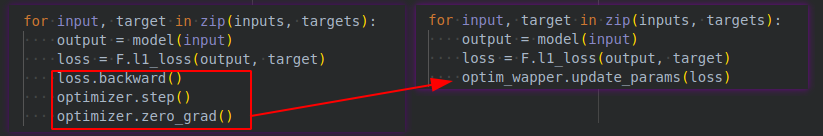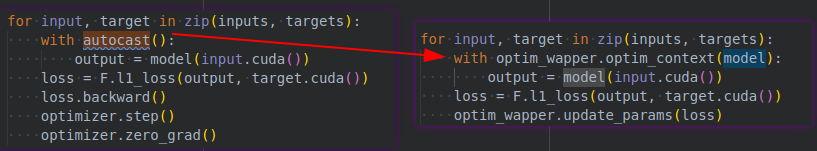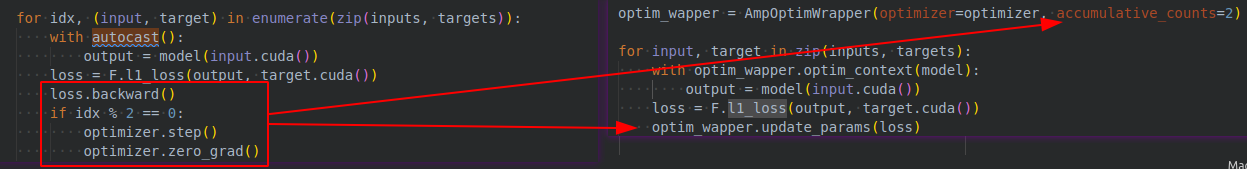Shortcuts

# OptimWrapper¶

In previous tutorials of runner and model, we have more or less mentioned the concept of OptimWrapper, but we have not introduced why we need it and what are the advantages of OptimWrapper compared to Pytorch’s native optimizer. In this tutorial, we will help you understand the advantages and demonstrate how to use the wrapper.

As its name suggests, OptimWrapper is a high-level abstraction of PyTorch’s native optimizer, which provides a unified set of interfaces while adding more functionality. OptimWrapper supports different training strategies, including mixed precision training, gradient accumulation, and gradient clipping. We can choose the appropriate training strategy according to our needs. OptimWrapper also defines a standard process for parameter updating based on which users can switch between different training strategies for the same set of code.

## OptimWrapper vs Optimizer¶

Now we use both the native optimizer of PyTorch and the OptimWrapper in MMEngine to perform single-precision training, mixed-precision training, and gradient accumulation to show the difference in implementations.

### Model training¶

1.1 Single-precision training with SGD in PyTorch

import torch
from torch.optim import SGD
import torch.nn as nn
import torch.nn.functional as F

inputs = [torch.zeros(10, 1, 1)] * 10
targets = [torch.ones(10, 1, 1)] * 10
model = nn.Linear(1, 1)
optimizer = SGD(model.parameters(), lr=0.01)

for input, target in zip(inputs, targets):
output = model(input)
loss = F.l1_loss(output, target)
loss.backward()
optimizer.step()


1.2 Single-precision training with OptimWrapper in MMEngine

from mmengine.optim import OptimWrapper

optim_wrapper = OptimWrapper(optimizer=optimizer)

for input, target in zip(inputs, targets):
output = model(input)
loss = F.l1_loss(output, target)
optim_wrapper.update_params(loss)The OptimWrapper.update_params achieves the standard process for gradient computation, parameter updating, and gradient zeroing, which can be used to update the model parameters directly.

2.1 Mixed-precision training with SGD in PyTorch

from torch.cuda.amp import autocast

model = model.cuda()
inputs = [torch.zeros(10, 1, 1, 1)] * 10
targets = [torch.ones(10, 1, 1, 1)] * 10

for input, target in zip(inputs, targets):
with autocast():
output = model(input.cuda())
loss = F.l1_loss(output, target.cuda())
loss.backward()
optimizer.step()


2.2 Mixed-precision training with OptimWrapper in MMEngine

from mmengine.optim import AmpOptimWrapper

optim_wrapper = AmpOptimWrapper(optimizer=optimizer)

for input, target in zip(inputs, targets):
with optim_wrapper.optim_context(model):
output = model(input.cuda())
loss = F.l1_loss(output, target.cuda())
optim_wrapper.update_params(loss)To enable mixed precision training, users need to use AmpOptimWrapper.optim_context which is similar to the autocast for enabling the context for mixed precision training. In addition, AmpOptimWrapper.optim_context can accelerate the gradient accumulation during the distributed training, which will be introduced in the next example.

3.1 Mixed-precision training and gradient accumulation with SGD in PyTorch

for idx, (input, target) in enumerate(zip(inputs, targets)):
with autocast():
output = model(input.cuda())
loss = F.l1_loss(output, target.cuda())
loss.backward()
if idx % 2 == 0:
optimizer.step()


3.2 Mixed-precision training and gradient accumulation with OptimWrapper in MMEngine

optim_wrapper = AmpOptimWrapper(optimizer=optimizer, accumulative_counts=2)

for input, target in zip(inputs, targets):
with optim_wrapper.optim_context(model):
output = model(input.cuda())
loss = F.l1_loss(output, target.cuda())
optim_wrapper.update_params(loss)We only need to configure the accumulative_counts parameter and call the update_params interface to achieve the gradient accumulation function. Besides, in the distributed training scenario, if we configure the gradient accumulation with optim_context context enabled, we can avoid unnecessary gradient synchronization during the gradient accumulation step.

The OptimWrapper also provides a more fine-grained interface for users to customize with their own parameter update logics.

• backward: Accept a loss dictionary, and compute the gradient of parameters.

• step: Same as optimizer.step, and update the parameters.

• zero_grad: Same as optimizer.zero_grad, and zero the gradient of parameters

We can use the above interface to implement the same logic of parameters updating as the Pytorch optimizer.

for idx, (input, target) in enumerate(zip(inputs, targets)):
with optim_wrapper.optim_context(model):
output = model(input.cuda())
loss = F.l1_loss(output, target.cuda())
optim_wrapper.backward(loss)
if idx % 2 == 0:
optim_wrapper.step()


We can also configure a gradient clipping strategy for the OptimWrapper.

# based on torch.nn.utils.clip_grad_norm_ method
optim_wrapper = AmpOptimWrapper(

optim_wrapper = AmpOptimWrapper(


### Get learning rate/momentum¶

The OptimWrapper provides the get_lr and get_momentum for the convenience of getting the learning rate and momentum of the first parameter group in the optimizer.

import torch.nn as nn
from torch.optim import SGD

from mmengine.optim import OptimWrapper

model = nn.Linear(1, 1)
optimizer = SGD(model.parameters(), lr=0.01)
optim_wrapper = OptimWrapper(optimizer)

print(optimizer.param_groups['lr'])  # 0.01
print(optimizer.param_groups['momentum'])  # 0
print(optim_wrapper.get_lr())  # {'lr': [0.01]}
print(optim_wrapper.get_momentum())  # {'momentum': }

0.01
0
{'lr': [0.01]}
{'momentum': }


Similar to the optimizer, the OptimWrapper provides the state_dict and load_state_dict interfaces for exporting and loading the optimizer states. For the AmpOptimWrapper, it can export mixed-precision training parameters as well.

import torch.nn as nn
from torch.optim import SGD
from mmengine.optim import OptimWrapper, AmpOptimWrapper

model = nn.Linear(1, 1)
optimizer = SGD(model.parameters(), lr=0.01)

optim_wrapper = OptimWrapper(optimizer=optimizer)
amp_optim_wrapper = AmpOptimWrapper(optimizer=optimizer)

# export state dicts
optim_state_dict = optim_wrapper.state_dict()
amp_optim_state_dict = amp_optim_wrapper.state_dict()

print(optim_state_dict)
print(amp_optim_state_dict)
optim_wrapper_new = OptimWrapper(optimizer=optimizer)
amp_optim_wrapper_new = AmpOptimWrapper(optimizer=optimizer)


{'state': {}, 'param_groups': [{'lr': 0.01, 'momentum': 0, 'dampening': 0, 'weight_decay': 0, 'nesterov': False, 'maximize': False, 'foreach': None, 'params': [0, 1]}]}
{'state': {}, 'param_groups': [{'lr': 0.01, 'momentum': 0, 'dampening': 0, 'weight_decay': 0, 'nesterov': False, 'maximize': False, 'foreach': None, 'params': [0, 1]}], 'loss_scaler': {'scale': 65536.0, 'growth_factor': 2.0, 'backoff_factor': 0.5, 'growth_interval': 2000, '_growth_tracker': 0}}


### Use multiple optimizers¶

Considering that algorithms like GANs usually need to use multiple optimizers to train the generator and the discriminator, MMEngine provides a container class called OptimWrapperDict to manage them. OptimWrapperDict stores the sub-OptimWrapper in the form of dict, and can be accessed and traversed just like a dict.

Unlike regular OptimWrapper, OptimWrapperDict does not provide methods such as update_prarms, optim_context, backward, step, etc. Therefore, it cannot be used directly to train models. We suggest implementing the logic of parameter updating by accessing the sub-OptimWarpper in OptimWrapperDict directly.

Users may wonder why not just use dict to manage multiple optimizers since OptimWrapperDict does not have training capabilities. Actually, the core function of OptimWrapperDict is to support exporting or loading the state dictionary of all sub-OptimWrapper and to support getting learning rates and momentums as well. Without OptimWrapperDict, MMEngine needs to do a lot of if-else in OptimWrapper to get the states of the OptimWrappers.

from torch.optim import SGD
import torch.nn as nn

from mmengine.optim import OptimWrapper, OptimWrapperDict

gen = nn.Linear(1, 1)
disc = nn.Linear(1, 1)
optimizer_gen = SGD(gen.parameters(), lr=0.01)
optimizer_disc = SGD(disc.parameters(), lr=0.01)

optim_wapper_gen = OptimWrapper(optimizer=optimizer_gen)
optim_wapper_disc = OptimWrapper(optimizer=optimizer_disc)
optim_dict = OptimWrapperDict(gen=optim_wapper_gen, disc=optim_wapper_disc)

print(optim_dict.get_lr())  # {'gen.lr': [0.01], 'disc.lr': [0.01]}
print(optim_dict.get_momentum())  # {'gen.momentum': , 'disc.momentum': }

{'gen.lr': [0.01], 'disc.lr': [0.01]}
{'gen.momentum': , 'disc.momentum': }


As shown in the above example, OptimWrapperDict exports learning rates and momentums for all OptimWrappers easily, and OptimWrapperDict can export and load all the state dicts in a similar way.

### Configure the OptimWapper in Runner¶

We first need to configure the optimizer for the OptimWrapper. MMEngine automatically adds all optimizers in PyTorch to the OPTIMIZERS registry, and users can specify the optimizers they need in the form of a dict. All supported optimizers in PyTorch are listed here.

Now we take setting up a SGD OptimWrapper as an example.

optimizer = dict(type='SGD', lr=0.01, momentum=0.9, weight_decay=0.0001)
optim_wrapper = dict(type='OptimWrapper', optimizer=optimizer)


Here we have set up an OptimWrapper with a SGD optimizer with the learning rate and momentum parameters as specified. Since OptimWrapper is designed for standard single precision training, we can also omit the type field in the configuration:

optimizer = dict(type='SGD', lr=0.01, momentum=0.9, weight_decay=0.0001)
optim_wrapper = dict(optimizer=optimizer)


To enable mixed-precision training and gradient accumulation, we change type to AmpOptimWrapper and specify the accumulative_counts parameter.

optimizer = dict(type='SGD', lr=0.01, momentum=0.9, weight_decay=0.0001)
optim_wrapper = dict(type='AmpOptimWrapper', optimizer=optimizer, accumulative_counts=2)


Note

If you are new to reading the MMEngine tutorial and are not familiar with concepts such as configs and registries, it is recommended to skip the following advanced tutorials for now and read other documents first. Of course, if you already have a good understanding of this prerequisite knowledge, we highly recommend reading the advanced part which covers:

1. How to customize the learning rate, decay coefficient, and other parameters of the model parameters in the configuration of OptimWrapper.

2. how to customize the construction policy of the optimizer.

Apart from the pre-requisite knowledge of the configs and the registries, it is recommended to have a thorough understanding of the native construction of PyTorch optimizer before starting the advanced tutorials.

PyTorch’s optimizer allows different hyperparameters to be set for each parameter in the model, such as using different learning rates for the backbone and head for a classification model.

from torch.optim import SGD
import torch.nn as nn

model = nn.ModuleDict(dict(backbone=nn.Linear(1, 1), head=nn.Linear(1, 1)))
optimizer = SGD([{'params': model.backbone.parameters()},
lr=0.01,
momentum=0.9)


In the above example, we set a learning rate of 0.01 for the backbone, while another learning rate of 1e-3 for the head. Users can pass a list of dictionaries containing the different parts of the model’s parameters and their corresponding hyperparameters to the optimizer, allowing for fine-grained adjustment of the model optimization.

In MMEngine, the optimizer wrapper constructor allows users to set hyperparameters in different parts of the model directly by setting the paramwise_cfg in the configuration file rather than by modifying the code of building the optimizer.

### Set different hyperparamters for different types of parameters¶

The default optimizer wrapper constructor in MMEngine supports setting different hyperparameters for different types of parameters in the model. For example, we can set norm_decay_mult=0 for paramwise_cfg to set the weight decay factor to 0 for the weight and bias of the normalization layer to implement the trick of not decaying the weight of the normalization layer as mentioned in the Bag of Tricks.

Here, we set the weight decay coefficient in all normalization layers (head.bn) in ToyModel to 0 as follows.

from mmengine.optim import build_optim_wrapper
from collections import OrderedDict

class ToyModel(nn.Module):
def __init__(self):
super().__init__()
self.backbone = nn.ModuleDict(
dict(layer0=nn.Linear(1, 1), layer1=nn.Linear(1, 1)))
OrderedDict(
linear=nn.Linear(1, 1),
bn=nn.BatchNorm1d(1)))

optim_wrapper = dict(
optimizer=dict(type='SGD', lr=0.01, weight_decay=0.0001),
paramwise_cfg=dict(norm_decay_mult=0))
optimizer = build_optim_wrapper(ToyModel(), optim_wrapper)

08/23 22:02:43 - mmengine - INFO - paramwise_options -- backbone.layer0.bias:lr=0.01
08/23 22:02:43 - mmengine - INFO - paramwise_options -- backbone.layer0.bias:weight_decay=0.0001
08/23 22:02:43 - mmengine - INFO - paramwise_options -- backbone.layer1.bias:lr=0.01
08/23 22:02:43 - mmengine - INFO - paramwise_options -- backbone.layer1.bias:weight_decay=0.0001
08/23 22:02:43 - mmengine - INFO - paramwise_options -- head.linear.bias:lr=0.01
08/23 22:02:43 - mmengine - INFO - paramwise_options -- head.linear.bias:weight_decay=0.0001
08/23 22:02:43 - mmengine - INFO - paramwise_options -- head.bn.weight:weight_decay=0.0
08/23 22:02:43 - mmengine - INFO - paramwise_options -- head.bn.bias:weight_decay=0.0


In addition to configuring the weight decay, paramwise_cfg of MMEngine’s default optimizer wrapper constructor supports the following hyperparameters as well.

lr_mult: Learning rate for all parameters.

decay_mult: Decay coefficient for all parameters.

bias_lr_mult: Learning rate coefficient of the bias (excluding bias of normalization layer and offset of the deformable convolution).

bias_decay_mult: Weight decay coefficient of the bias (excluding bias of normalization layer and offset of the deformable convolution).

norm_decay_mult: Weight decay coefficient for weights and bias of the normalization layer.

flat_decay_mult: Weight decay coefficient of the one-dimension parameters.

dwconv_decay_mult: Decay coefficient of the depth-wise convolution.

bypass_duplicate: Whether to skip duplicate parameters, default to False.

dcn_offset_lr_mult: Learning rate of the deformable convolution.

### Set different hyperparamters for different model modules¶

In addition, as shown in the PyTorch code above, in MMEngine we can also set different hyperparameters for any module in the model by setting custom_keys in paramwise_cfg.

If we want to set the learning rate and the decay coefficient to 0 for backbone.layer0, and set the learning rate to 0.001 for the rest of the modules in the backbone. At the same time, we want to keep all the learning rate to 0.001 for the head module. We can do it in this way:

optim_wrapper = dict(
optimizer=dict(type='SGD', lr=0.01, weight_decay=0.0001),
paramwise_cfg=dict(
custom_keys={
'backbone.layer0': dict(lr_mult=0, decay_mult=0),
'backbone': dict(lr_mult=1),
}))
optimizer = build_optim_wrapper(ToyModel(), optim_wrapper)

08/23 22:02:43 - mmengine - INFO - paramwise_options -- backbone.layer0.weight:lr=0.0
08/23 22:02:43 - mmengine - INFO - paramwise_options -- backbone.layer0.weight:weight_decay=0.0
08/23 22:02:43 - mmengine - INFO - paramwise_options -- backbone.layer0.weight:lr_mult=0
08/23 22:02:43 - mmengine - INFO - paramwise_options -- backbone.layer0.weight:decay_mult=0
08/23 22:02:43 - mmengine - INFO - paramwise_options -- backbone.layer0.bias:lr=0.0
08/23 22:02:43 - mmengine - INFO - paramwise_options -- backbone.layer0.bias:weight_decay=0.0
08/23 22:02:43 - mmengine - INFO - paramwise_options -- backbone.layer0.bias:lr_mult=0
08/23 22:02:43 - mmengine - INFO - paramwise_options -- backbone.layer0.bias:decay_mult=0
08/23 22:02:43 - mmengine - INFO - paramwise_options -- backbone.layer1.weight:lr=0.01
08/23 22:02:43 - mmengine - INFO - paramwise_options -- backbone.layer1.weight:weight_decay=0.0001
08/23 22:02:43 - mmengine - INFO - paramwise_options -- backbone.layer1.weight:lr_mult=1
08/23 22:02:43 - mmengine - INFO - paramwise_options -- backbone.layer1.bias:lr=0.01
08/23 22:02:43 - mmengine - INFO - paramwise_options -- backbone.layer1.bias:weight_decay=0.0001
08/23 22:02:43 - mmengine - INFO - paramwise_options -- backbone.layer1.bias:lr_mult=1
08/23 22:02:43 - mmengine - INFO - paramwise_options -- head.linear.weight:lr=0.001
08/23 22:02:43 - mmengine - INFO - paramwise_options -- head.linear.weight:weight_decay=0.0001
08/23 22:02:43 - mmengine - INFO - paramwise_options -- head.linear.weight:lr_mult=0.1
08/23 22:02:43 - mmengine - INFO - paramwise_options -- head.linear.bias:lr=0.001
08/23 22:02:43 - mmengine - INFO - paramwise_options -- head.linear.bias:weight_decay=0.0001
08/23 22:02:43 - mmengine - INFO - paramwise_options -- head.linear.bias:lr_mult=0.1
08/23 22:02:43 - mmengine - INFO - paramwise_options -- head.bn.weight:lr=0.001
08/23 22:02:43 - mmengine - INFO - paramwise_options -- head.bn.weight:weight_decay=0.0001
08/23 22:02:43 - mmengine - INFO - paramwise_options -- head.bn.weight:lr_mult=0.1
08/23 22:02:43 - mmengine - INFO - paramwise_options -- head.bn.bias:lr=0.001
08/23 22:02:43 - mmengine - INFO - paramwise_options -- head.bn.bias:weight_decay=0.0001
08/23 22:02:43 - mmengine - INFO - paramwise_options -- head.bn.bias:lr_mult=0.1


The state dictionary of the above model can be printed as the following:

for name, val in ToyModel().named_parameters():
print(name)

backbone.layer0.weight
backbone.layer0.bias
backbone.layer1.weight
backbone.layer1.bias


Each field in custom_keys is defined as follows.

1. 'backbone': dict(lr_mult=1): Set the learning rate of the parameter whose name is prefixed with backbone to 1.

2. 'backbone.layer0': dict(lr_mult=0, decay_mult=0): Set the learning rate of the parameter with the prefix backbone.layer0 to 0 and the decay coefficient to 0. This configuration has a higher priority than the first one.

3. 'head': dict(lr_mult=0.1): Set the learning rate of the parameter whose name is prefixed with head to 0.1.

### Customize optimizer construction policies¶

Like other modules in MMEngine, the optimizer wrapper constructor is also managed by the registry. We can customize the hyperparameter policies by implementing custom optimizer wrapper constructors.

For example, we can implement an optimizer wrapper constructor called LayerDecayOptimWrapperConstructor that automatically set decreasing learning rates for layers of different depths of the model.

from mmengine.optim import DefaultOptimWrapperConstructor
from mmengine.registry import OPTIM_WRAPPER_CONSTRUCTORS
from mmengine.logging import print_log

@OPTIM_WRAPPER_CONSTRUCTORS.register_module(force=True)
class LayerDecayOptimWrapperConstructor(DefaultOptimWrapperConstructor):

def __init__(self, optim_wrapper_cfg, paramwise_cfg=None):
super().__init__(optim_wrapper_cfg, paramwise_cfg=None)
self.decay_factor = paramwise_cfg.get('decay_factor', 0.5)

super().__init__(optim_wrapper_cfg, paramwise_cfg)

def add_params(self, params, module, prefix='' ,lr=None):
if lr is None:
lr = self.base_lr

for name, param in module.named_parameters(recurse=False):
param_group = dict()
param_group['params'] = [param]
param_group['lr'] = lr
params.append(param_group)
full_name = f'{prefix}.{name}' if prefix else name
print_log(f'{full_name} : lr={lr}', logger='current')

for name, module in module.named_children():
chiled_prefix = f'{prefix}.{name}' if prefix else name
params, module, chiled_prefix, lr=lr * self.decay_factor)

class ToyModel(nn.Module):

def __init__(self) -> None:
super().__init__()
self.layer = nn.ModuleDict(dict(linear=nn.Linear(1, 1)))
self.linear = nn.Linear(1, 1)

model = ToyModel()

optim_wrapper = dict(
optimizer=dict(type='SGD', lr=0.01, weight_decay=0.0001),
paramwise_cfg=dict(decay_factor=0.5),
constructor='LayerDecayOptimWrapperConstructor')

optimizer = build_optim_wrapper(model, optim_wrapper)

08/23 22:20:26 - mmengine - INFO - layer.linear.weight : lr=0.0025
08/23 22:20:26 - mmengine - INFO - layer.linear.bias : lr=0.0025
08/23 22:20:26 - mmengine - INFO - linear.weight : lr=0.005
08/23 22:20:26 - mmengine - INFO - linear.bias : lr=0.005


When add_params is called for the first time, the params argument is an empty list and the module is the ToyModel instance. Please refer to the Optimizer Wrapper Constructor Documentation for detailed explanations on overloading.

Similarly, if we want to construct multiple optimizers, we also need to implement a custom constructor.

@OPTIM_WRAPPER_CONSTRUCTORS.register_module()
class MultipleOptimiWrapperConstructor:
...


The hyperparameters in the optimizer can only be set to a fixed value at the time it is constructed, and you cannot adjust parameters such as the learning rate during training by just using the optimizer wrapper. In MMEngine, we have implemented a parameter scheduler that allows the tuning of parameters during training. For the usage of the parameter scheduler, please refer to the Parameter Scheduler

© Copyright 2022, mmengine contributors. Revision c863e8b1.

Built with Sphinx using a theme provided by Read the Docs.
Versions
latest
stable
v0.8.5
v0.8.4
v0.8.3
v0.8.2
v0.8.1
v0.8.0
v0.7.4
v0.7.3
v0.7.2
v0.7.1
v0.7.0
v0.6.0
v0.5.0
v0.4.0
v0.3.0
v0.2.0